• C语言-顺序栈实现十进制转换为二进制-八进制-十六进制
• 栈的应用举例，由于没给出完整源码，自己写了一个比较完整的
• C语言实现十进制转换成二十六进制的转换，代码已经经过调试可以使用，放心下载！C语言
• //题目：八进制转换为十进制 #include<stdio.h> #include<math.h> int main() { int i=0,n,tmp,sum=0; scanf("%d",&n); while(n) { tmp=n%10; n=n/10; sum+=tmp*pow(8,i); i++;...
基本思想: 八进制转十进制，二进制转十进制都一样的道理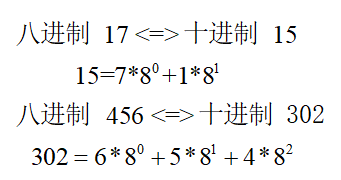八进制 --> 十进制
//【程序48】
//题目：八进制-->十进制
#include<stdio.h>
#include<math.h>
int main()
{
int i=0,n,tmp,sum=0;
scanf("%d",&n);
while(n)
{
tmp=n%10;
n=n/10;
sum+=tmp*pow(8,i);
i++;
}
printf("%d",sum);
return 0;
}

二进制 --> 十进制
#include<stdio.h> //二进制-->十进制
#include<math.h>
int main()
{
int i=0,n,tmp,sum=0;
scanf("%d",&n);
while(n)
{
tmp=n%10;
n=n/10;
sum+=tmp*pow(2,i);
i++;
}
printf("%d",sum);
return 0;
}

十进制 --> 二进制
#include<stdio.h>  //十进制-->二进制
int main()
{
int i=0,n,str;
scanf("%d",&n);
while(n)
{
str[i]=n%2;
n=n/2;
i++;
}
for(int j=i-1;j>=0;j--)
printf("%d",str[j]);
return 0;
}

十进制 --> 八进制
#include<stdio.h>  //十进制-->八进制
int main()
{
int i=0,n,str;
scanf("%d",&n);
while(n)
{
str[i]=n%8;
n=n/8;
i++;
}
for(int j=i-1;j>=0;j--)
printf("%d",str[j]);
return 0;
}

十六进制 --> 十进制
#include<stdio.h> //十六进制-->十进制 eg. (十六进制)AB-->171(十进制)
#include<math.h>                                  //ABCD-->43981
int main()
{
int i,j,k=0;
long int sum=0,tmp;
char str,a;
gets(str);
for(i=0;str[i]!='\0';i++)
{
if(str[i]>='0'&&str[i]<='9')
a[i]=str[i]-'0';
if(str[i]>='a'&&str[i]<='z')
a[i]=str[i]-'a'+1+9;
if(str[i]>='A'&&str[i]<='Z')
a[i]=str[i]-'A'+1+9;
}
for(j=i-1;j>=0;j--)
{
tmp=a[j]*pow(16,k);
sum+=tmp;
k++;
}
printf("%ld",sum);
return 0;
}
//#include<stdio.h>         //方法二：参看大佬的解法
//int main()                //16-->10进制
//{
//	int num = 0;
//    printf("输入一个十六进制数: ");
//    scanf("%x", &num);
//    printf("十六进制数 %x 转换为十进制为 %d\n", num, num);
//	return 0;
//}

十进制 --> 十六进制
#include<stdio.h>
int main()
{
int n,i=0,j;
char ten,str="0123456789ABCDEF";  //10进制-->16进制
scanf("%d",&n);                           //46-->2E; 59-->3B
while(n)                                  //46= 2*16^1 + 14*16^0
{
ten[i++]=str[n%16];
n=n/16;
}
for(j=i-1;j>=0;j--)
printf("%c",ten[j]);
return 0;
}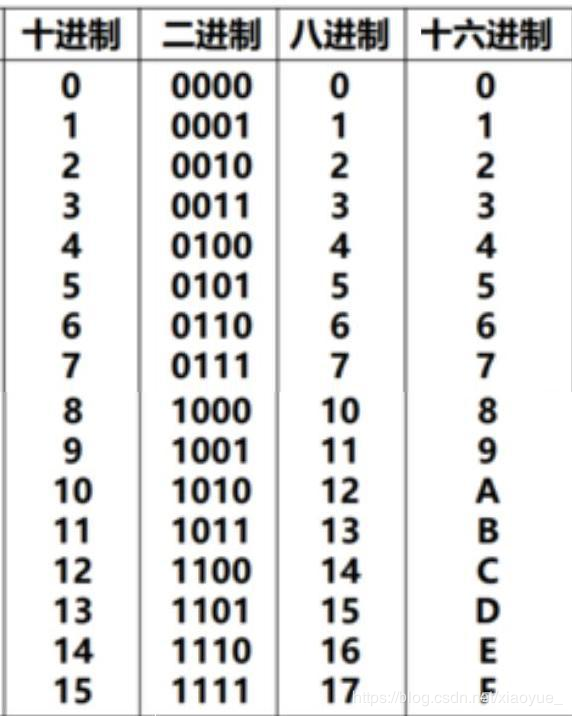展开全文c语言
• 样例输入：12 样例输出：014 #include<bits/stdc++.h> using namespace std; int l=0,ans= {0}; void change(int x) { if(x<8) { ans[l++]=x; for(int i=l-1; i>... change(x/=8
样例输入：12 样例输出：014
#include<bits/stdc++.h>
using namespace std;
int l=0,ans= {0};
void change(int x)
{
if(x<8)
{
ans[l++]=x;
for(int i=l-1; i>=0; i--)
{
cout<<ans[i];
}
return;
}
else
{
ans[l++]=x%8;
}
change(x/=8);
}
int main()
{
int num;
cin>>num;
cout<<0;
change(num);
return 0;
}
展开全文c++ c语言 c#
• 课程的随堂作业，C语言的，用dev就能运行，萌新代码，勿喷，仅仅帮助不想写作业的朋友方便一下，反正老师也不会仔细检查的C语言
• 常用的进制有二进制，八进制十进制，十六进制。 二.将整数转换成十六进制 二.一 将int整数转换成十六进制（一） 先写一个主方法，接下来，所有的方法都是通过main 方法来调用的。 不用Junit测试。 做静态的方法，...
一. 进制转换
在生活和实际开发中，进制转换是一个常考的知识点。 虽然Java开发者已经对其进行了封装，但还是要学会自己编写相应的进制转换器的。 常用的进制有二进制，八进制，十进制，十六进制。
二.将整数转换成十六进制
二.一 将int整数转换成十六进制（一）
先写一个主方法，接下来，所有的方法都是通过main 方法来调用的。 不用Junit测试。 做静态的方法，类似于工具的形式。
public static void main(String []args){
System.out.println(printOX1(1024));
}

先用最简单的方法进行写程序。 求十六进制，就是将这个数字不断的除以16，然后得到余数。 去判断这个余数的大小，如果是0 ~ 9 ，那么就用数字0 ~ 9 来表示，如果是10 ~ 15 ,那么 就用字符’A’ ~ ‘F’ 或者小写的 ‘a’ ~ ‘f’ 来表示。 然后，用商来替换这个数字，继续进行循环。 最简单的方式，先用一个字符串来接收转换后的进制结果。要循环八次。 为什么用8呢，因为int型是4个字节，十六进制最大用四个字符来表示。 所以，最大是4*8/4=8 个长度。
public static String printOX1(int num){
//1. 判断，如果是0的话，就直接返回0
if(num==0){
return "0";
}
//2.16进制的话，存储int型，可以存储4*32/16=8 个长度。
StringBuilder sb=new StringBuilder();
for (int i = 0; i <8; i++) {
//3. 将这些数字除以16,得到余数。 求的是低四位。
int temp=num&15;
if(temp>9){
sb.append(temp-10+'A');
}else{
sb.append(temp);
}
num=num>>>4; //除以16,得到商。 将商当成除数。
}
return sb.toString();
}

上面这个运行输出后，结果是: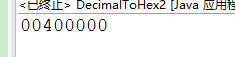这个顺序是颠倒的，并且还有多余的0存在。
二.二 将int整数转换成十六进制（二）
改进: 根据余数来求数字和字符，其实是可以转换成数组的。 利用数组的查表法进行相应的改变。 将余数放入到数组的下标索引上，就可以取出相应的表示字符。
public static String printOX2(int num){
//1. 判断，如果是0的话，就直接返回0
if(num==0){
return "0";
}
// 定义一个字符数组，这个索引与十六进制的表示方式一样。
char [] hexs={'0','1','2','3','4','5','6','7','8','9',
'A','B','C','D','E','F'};
StringBuilder sb=new StringBuilder();
for (int i = 0; i <8; i++) {
int temp=num&15;
sb.append(hexs[temp]); // 根据下标去求数组中查询相应的值。
num=num>>>4;
}
return sb.toString();
}

运行后，结果仍然是: 00400000, 顺序颠倒，有零。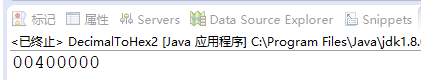二.三 将int整数转换成十六进制（三）
利用数组的方式去解决。 将得到的这个值，倒序放入到一个数组中，就是将004 00000 倒序放入数组中。 那么 此时 a=0,a=0,… … a=0, a=4, a=0,a=0; 然后将这个数组从非零的索引位置处进行输出即可。 要记录下这个非零的索引位置。
public static String printOX3(int num){
//1. 判断，如果是0的话，就直接返回0
if(num==0){
return "0";
}
//2.16进制的话，存储int型，可以存储4*32/16=8 个长度。
char [] hexs={'0','1','2','3','4','5','6','7','8','9',
'A','B','C','D','E','F'};
char [] tabs=new char;
StringBuilder sb=new StringBuilder();
int pos=tabs.length;
while (num>0) {  //不知道要运行几次。
//3. 将这些数字除以16,得到那个要转换的值。
int temp=num&15;
tabs[--pos]=hexs[temp];  //上面用的是长度，这里用--pos. 要注意，索引越界的问题。
num=num>>>4;
}
for(int i=pos;i<8;i++){
sb.append(tabs[i]);
}
return sb.toString();
}

运行之后，就是: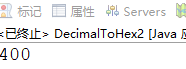正确的十六进制数字。
二.四 将int整数转换成十六进制（四）
利用String 的format 格式化方法进行求解。 格式信息是 %x. x为十六进制。
    String.format("%x",1024);   // 是String 类型

二.五 将int整数转换成十六进制（五）
Java底层开发人员，封装了一个方法。 可以自动将数字进行转换成十六进制。 开发中，推荐使用这一个。
	Integer.toHexString(1024)    //转换成十六进制。 二进制，八进制，也有类似的方法。

三. 十进制转其他的进制
只支持常见的二进制，八进制，十六进制。 这种方式，暂时不支持其他的进制。后面有其他的方法会慢慢支持的。
public static void main(String []args){
int num=1024;
System.out.println("十六进制:"+toHex(num)); //转换成十六进制
System.out.println("二进制:"+toBinary(num)); //转换成二进制
System.out.println("八进制:"+toOct(num)); //转换成八进制
}

其中，十六进制的方法是:
public static String toHex(int num) {
return  toTrave(num,15,4); //基数是15，右移4位。
}

虽然二进制和八进制，已经可以证明，肯定是数字型的。但由于位数相比较十进制较多，用int 或者long接收的话，都有可能造成溢出。 所以，不建议用long 或者int 接收 。 直接用字符串接收，是数字型的字符串。 其中，八进制的方法是:
public static String toOct(int num) {
}

其中，二进制的方法是:
public static String toBinary(int num) {
}

共同的方法是 toTrave()的定义是:
public static String toTrave(int num,int base,int moveIndex){
//1. 判断，如果是0的话，就直接返回0
if(num==0){
return "0";
}
//2.16进制的话，存储int型，可以存储4*32/16=8 个长度。
char [] hexs={'0','1','2','3','4','5','6','7','8','9',
'A','B','C','D','E','F'};
char [] tabs=new char;
StringBuilder sb=new StringBuilder();
int pos=tabs.length;
while (num>0) {  //不知道要运行几次。
//3. 将这些数字除以基数,得到那个要转换的值。
int temp=num&base;
tabs[--pos]=hexs[temp];  //上面用的是长度，这里用--pos. 要注意，索引越界的问题。
num=num>>>moveIndex;
}
for(int i=pos;i<8;i++){
sb.append(tabs[i]);
}
return sb.toString();
}

与上面的printOX3（） 方法是一样的。 只是将15，和>>>4 的4 转换成局部变量而已。
四. String.format 方法的支持
		System.out.println(String.format("%x",1024));//十六进制
System.out.println(String.format("%o",1024)); //八进制
System.out.println(String.format("%d",1024)); //十进制
System.out.println(String.format("%b",1024)); //%b,并不是二进制，而是会输出true. 非空为true

五. Integer 的toString() 方法封装
		System.out.println(Integer.toBinaryString(1024)); //二进制
System.out.println(Integer.toOctalString(1024)); //八进制
System.out.println(Integer.toHexString(1024)); //十六进制

如果要转换成其他进制的话，用toString(十进制数字num,要转换的进制位) 的方法。
System.out.println(Integer.toString(1024,7));   //转换成七进制数
System.out.println(Integer.toString(1024,2));  //当然，也可以用它转换成二进制数。

五. Integer 的parseInt(“数字字符串”,“原先的进制”) 和valueOf(“数字字符串”,原先的进制) 方法封装
这两个方法，都是转换成十进制的。
System.out.println(Integer.parseInt("400",16)); //将16进制的400转换成十进制
System.out.println(Integer.valueOf("400",8));//将8进制的400转换成十进制

谢谢!!
展开全文• JAVA进制转换,十进制转换为八进制 package cn.wf.test; import java.util.Stack; /** * 进制转换,十进制转换为八进制 * @author wf * */ public class baseConversion { public static void main(String[] ...
JAVA进制转换,十进制转换为八进制
package cn.wf.test;

import java.util.Stack;

/**
* 进制转换,十进制转换为八进制
* @author wf
*
*/

public class baseConversion {

public static void main(String[] args) {
Basc(20);

}
public static void Basc(int i) {
Stack s = new Stack();
while (i>0) {
s.push(i%8+"");
i=i/8;
}
while(!s.isEmpty())System.out.print((String)s.pop());
}

}

展开全文• #include <iostream.h> //十进制转换成二进制数字 void fun_1(int n) { if(n) cout;... } } //十进制数字转换八进制数字 void fun_2(int n) { if(n) cout; if(n>=8) { fun_2(n/8; cout; } } //十
• 该资源是用于将十进制转换为八进制数的程序，编程语言c#，程序简单易懂，便于实用。
• 问题描述：使用C语言编程实现八进制转换为十进制程序源码： #include&lt;stdio.h&gt; void main() { char *p,s;int n; p=s; gets(p); n=0; while(*(p)!='\0') {n=n*8+*p-'0'; p++;} printf("...C语言编程
• Here you will get python program to convert decimal ... 在这里，您将获得将十进制转换为二进制，八进制和十六进制的python程序。 Python provides inbuilt functions for conversion of one number system to a...python 深度学习 tensorflow c++
• 我们处理的整数通常用十进制表示，在计算机内存中是以二进制补码形式存储，但通常二进制表示的整数比较长，为了便于在程序设计过程中理解和处理数据，通常采用八进制和十六进制，缩短了二进制补码表示的整数，但保持...c语言
• 主要介绍了python十进制和二进制的转换方法(含浮点数)，小编觉得挺不错的，现在分享给大家，也给大家做个参考。一起跟随小编过来看看吧
• I have this little homework assignment and I needed to convert decimal to octal and then octal to decimal. I did the first part and can not figure out the second to save my life. The first part went l...
• 输入一个十进制数可以转化成二进制，八进制，十六进制。.java格式的右键编辑可以打开。
• 用顺序表实现数值转换（此程序为十进制转换八进制） #include <stdio.h> #include <stdlib.h> #define OK 1 #define ERROR 0 #define OVERFLOW -2 #define STACKSIZE 100 #define ...
• 输入2个十进制整数，分别按照十六进制，十进制进制表格式输出。 【样例输入】 12 23 【样例输出】 0xc 12 014 0x17 23 027 【样例说明】 每一项的输出宽度均8位，左对齐。 #include <...C++
• #include&lt;iostream&gt; #define MaxSize 100 ...//进制转换栈的操作 using namespace std; typedef int ElemType; typedef int Status; typedef struct StackNode { ElemType data; struct...c 数据结构
• ## 十进制转换成八进制（C++）

万次阅读 多人点赞 2019-07-20 16:45:39
用递归算法，把任一给定的十进制正整数转换八进制数输出。 Input 输入一个正整数，表示需要转换十进制数。 Output 输出一个正整数，表示转换之后的八进制的数。 Sample Input 15 Sample Output 17 HINT...
• c语言栈实现十进制转换为二进制，八进制和十六进制 写一个程序,将输入的十进制数据M 转换为八进制数据M8,将其调试通过.在此基础上修改程序,实现十进制数据M 向N 进制(2或8或16)的转换. #include<stdio.h> #...
• 十进制数N和其它d进制数的转换是计算机实现计算的基本问题，其解决方案很多，其中最简单方法基于下列原理：即除d取余法。
• 编写函数，其功能把一个十进制转换为其对应的八进制数。程序读入一个十进制数，调用该函数实现数制转换后，输出对应的八进制数。 样例输入 9274 样例输出 22072 样例输入 18 样例输出 ...
• C语言十进制转换为八进制C语言十进制转换为八进制完整源码 C语言十进制转换为八进制完整源码 #include <stdio.h> void decimal2Octal(long decimalnum); int main() { long decimalnum; printf(...
• 使用程序来实现十进制转换为不同的进制，那么关键在于如何将每次的余数保存并正确输出。要知道余数最后是按照倒序输出的，所以这个是一个问题点。（进制的转换方法计算在我的上一篇文章中） #include <stdio.h&...
• 数制转换器 该程序会将给定的数字转换为其他数字系统 二进制 奥卡泰尔 十进制 十六进制 里程碑 创建函数 在适当的地方调用函数。 显示结果Java
• printf("输入要转换为八进制数的十进制数："); scanf("%d",&n); OctConversion(stack,n); printf("\n"); printf("输入要转换为十六进制的十进制数："); scanf("%d",&m); HexConversion(stack,m); printf("\n"); ...指针 数据结构 链表 算法 c语言
• 数据结构 study 6： 栈 实现 十进制 转换为 8进制数据结构 c语言...